# Coefficients in the linear combination of a k-form

• A
• Maxi1995
Differential forms are linear transformations between vector spaces- The exterior derivative is a linear operation between vector spaces- The exterior derivative of a function is the derivative of the function at a point where the function has an infinitesimal change of coordinates- The exterior derivative is a linear operation between vector spaces, and it can be decomposed into the product of a linear operator and a vector- The exterior derivative is a vector, and it has a gradientf

#### Maxi1995

Hello,
We defined a k-form on a smooth manifold M as a transfromation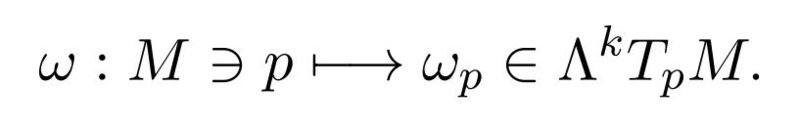Where the right space is the one of the alternating k-linear forms over the tangent space in p.
If we suppose we know, that we get a basis of this space by using the wedge-product and a basis of the dual space, then we might get for a given form: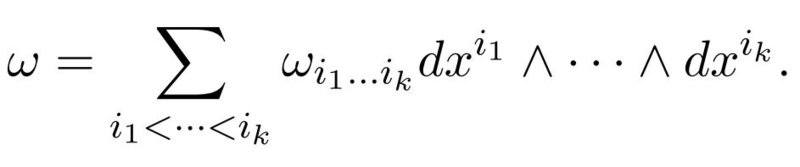Can someone explain to me what the coefficient in this linear combination is? I know that they are functions and in some cases scalars. But I don't know the cases. According to the definition at the beginning I suppose, that they are funtions for a given p and become scalars if we have argument values of the tangent space. Is this correct?
Greetings
Maxi

#### Attachments

You have ##\omega \in \Lambda^k TM## which is a vector space with basis vectors ##dx^{i_1}\wedge \ldots \wedge dx^{i_k}##. Thus the ##\omega_{i_1 \ldots i_k}## are scalars, the coefficients in the linear combination which represents ##\omega ## according to this basis.

The evaluation ##p \longmapsto \omega_p## at a certain point affects only the differential forms, resp. the function those forms apply to, not the scalar coefficients.

E.g. if ##k=1## and ##\omega = 2dx## we get ##p \longmapsto \omega_p = (f \longmapsto 2df_p)## or ##f(p+v)=f(p)+df_p\cdot v + o(v)=f(p)+\frac{1}{2}\omega_p(f)\cdot v+o(v)##. The coefficient is not affected.

What fresh says is of course exactly right, but in one special case, you can say more about these coefficients. if you have a set of k one- forms, each written in terms of the n basic one forms dx1,...,dxn, then each of your one - forms needs n coefficients. If you wedge together those k one - forms, you get a k-form expressible in terms of the standard coordinate k-forms, as you have written, with some coefficients you have asked about. Those cofficients in this case are exactly all k by k sub determinants of the n by k matrix of coefficients you began with, the ones expressing the k arbitrary one - forms in terms of the n standard coordinate one-forms. Note there are exactly "n choose k" such k by k subdeterminants.

Indeed if you prove independently that the space of n-forms (at one point) is one dimensional, then one way to define the determinant of a matrix is as the coefficient needed to write the wedge product of its rows in terms of the standard n-form. More abstractly, a linear endomorphism of an n dimensional vector space induces a linear endomorphism of its space of n-linear alternating functions, which must be multiplication by a scalar, which scalar is then defined as the determinant of the original endomorphism.

Hello,
@ fresh_42
What is f in your notation? Is it a curve?
I understand what you said about the elements of the basis, thus to say that they are just scalars. But I found in a Wikipedia article (https://en.wikipedia.org/wiki/Differential_form#Differential_calculus) that we might find a linear combination where these factors are smooth functions from $$U \subset \mathbb{R}^n \rightarrow \mathbb{R}$$. Because of this I'm going to add more detail the context in which my question arose.
We defined the outer derivative on $$\mathbb{R}^n$$ as follows: Let $$w$$ be a k-form on a open set U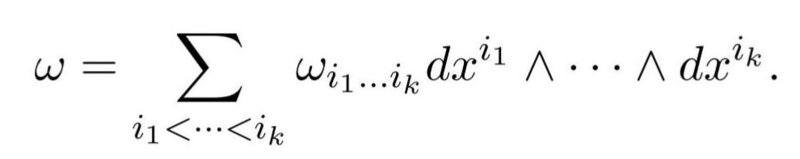Then we define the exteriour derivative by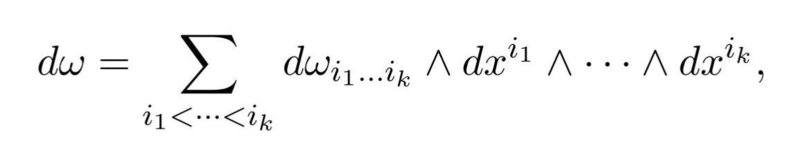where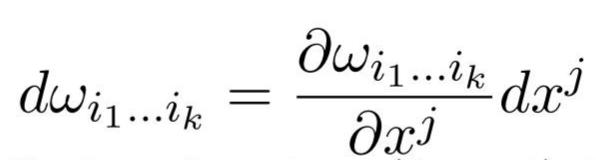is the (normal) differential of the function $$w_{i_1 \cdots i_k}$$ (Please note that we used here the Einstein notation, so we have a sum over j.).
As you said I expected to be the coefficients scalars, but in this case they are just smooth functions, what puzzled me. Can you explain that to me?
@mathwonk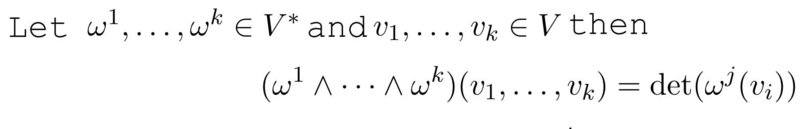Where $$V^*$$ is the dual space and j counts the lines and i the columns. What do you mean by subdeterminants?
Best wishes
Maxi

#### Attachments

What is f in your notation? Is it a curve?
A smooth function the differential form ##\omega ## applies to, so in general no curve, simply a variable.

The moment you write ##d\omega## you do no longer consider a certain and thus fixed vector in ##\Lambda^k## but investigate what happens by infinitesimal changes of coordinates. You switch from ## \vec{v}=v_0\vec{e}_0## to ##\vec{v}(t)=v_0(t)\vec{e}_0##. It is literally the transition from algebra to calculus. Of course now you will have to say in which way these coordinates totter, i.e. what is ##t \mapsto v_0(t)## or in general and local coordinates again, what is ##d\omega_{i_1\ldots i_k} = \sum_{j}\dfrac{\partial \omega_{i_1\ldots i_k}}{\partial x^j}dx^j\,.##

If you want to know, why this might confusing you, have a look at the 10 items list at the end of section 1 herein:
https://www.physicsforums.com/insights/journey-manifold-su2mathbbc-part/

I'm not sure whether it will confuses you further or actually be of help, but at least it might serve as a quick reference guide (5 parts):
https://www.physicsforums.com/insights/the-pantheon-of-derivatives-i/

Last edited:
Hello,
I have another question in this context. Let us say we want to talk about the function as object, thus to say the function itself as element of $$\Lambda ^k V.$$ We derived the coefficients in our proofs by using the smooth k-form itself. We set the coefficients as $$w_{i_1...i_k}=w(e_{i_1},...,e_{i_k})$$. From this and the fact that we made other proofs in $$\Lambda^n V$$ where V was n-dimensional and $$w$$ had the form $$w= f dx_1\wedge...\wedge dx_n$$ with f a smooth function from $$U \subset \mathbb{R}^n \rightarrow \mathbb{R}.$$ I'm still a little bit puzzled, but think this might be caused by vieweing the function as object. Is that right?